# Pyplot简介

matplotlib.pyplot 是一个命令风格的函数集合，这使得 matplotlib 工作起来和MATLAB很相似。每一个 pyplot 函数都会使图形发生一些变化，例如：创建一幅图形、在一幅图中创建一个绘图区域、在绘图区域中绘制一些线、使用标签对图形进行修饰，等等。在matplotlib.pyplot中，各种各样的状态通过调用不同的函数被保存下来，因此，matplotlib 能够持续地对例如：当前的图形和绘图区域、并将绘图函数作用到当前轴上（请注意，这里和文档中大多数地方的“轴”指的是图形的坐标系部分，而不是严格数学术语中的多个轴）。
使用 pyplot 生成一个可视化效果非常快：

import matplotlib.pyplot as plt
plt.plot([1,2,3,4])
plt.ylabel('some numbers')
plt.show()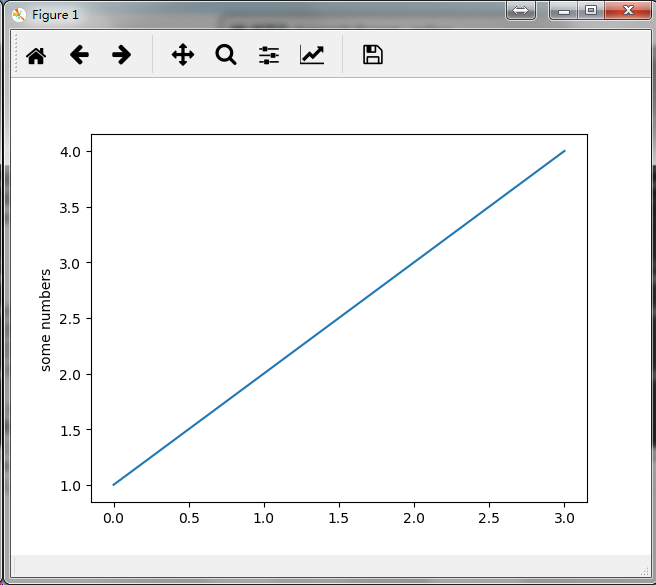plot() 是一个多功能的命令，能够接收任意数量的参数，例如：你可以使用如下命令来绘制与X相对的Y的图形。

plt.plot([1, 2, 3, 4], [1, 4, 9, 16])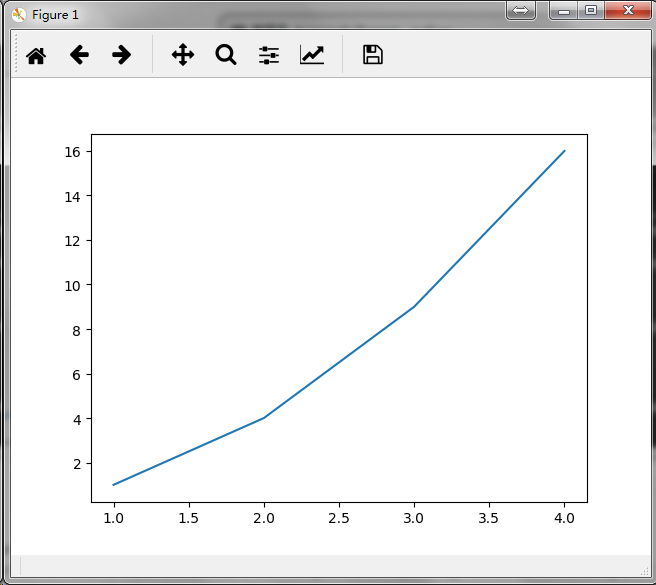# 格式化图形样式

plot() 还为每一对参数X和Y提供了一个可选的第三个格式化字符串参数用来指定图形的颜色和线型。该格式化字符串的字母和符号均来自于MATLAB，由一个代表颜色的字符串和一个代表线型的字符串组合而成。默认使用的格式化字符串是’b-‘（表示蓝色实线）。
如果想要使用红色的圆点来绘制上例中的图形，你可以这么写：

plt.plot([1, 2, 3, 4], [1, 4, 9, 16], 'ro')
plt.axis([0, 6, 0, 20])
plt.show()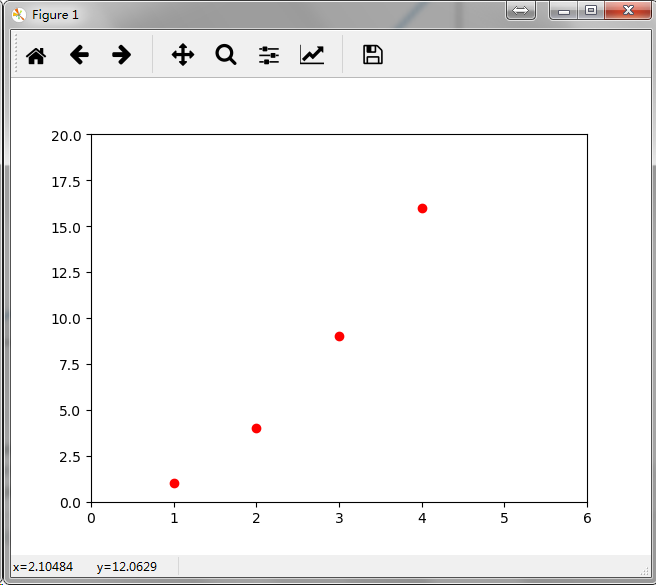你可以查看 plot() 的文档来获取关于线的样式和格式化字符串的完整列表。
上例中的 axis() 命令接收了 [xmin, xmax, ymin, ymax] 列表作为参数来指定了各个轴的视口大小。但如果 Matplotlib 被限制成只能和 list 一起使用 ，那么它在数值处理上将显得几乎毫无用处 。通常，我们都会使用 numpy 数组，事实上，所有的序列都会在 Matplotlib 内部被转化成 numpy 数组。下面的例子展示了如何在一条命令里使用  numpy 数组绘制出几条样式不同的线：

import numpy as np

# evenly sampled time at 200ms intervals
t = np.arange(0., 5., 0.2)

# red dashes, blue squares and green triangles
plt.plot(t, t, 'r--', t, t**2, 'bs', t, t**3, 'g^')
plt.show()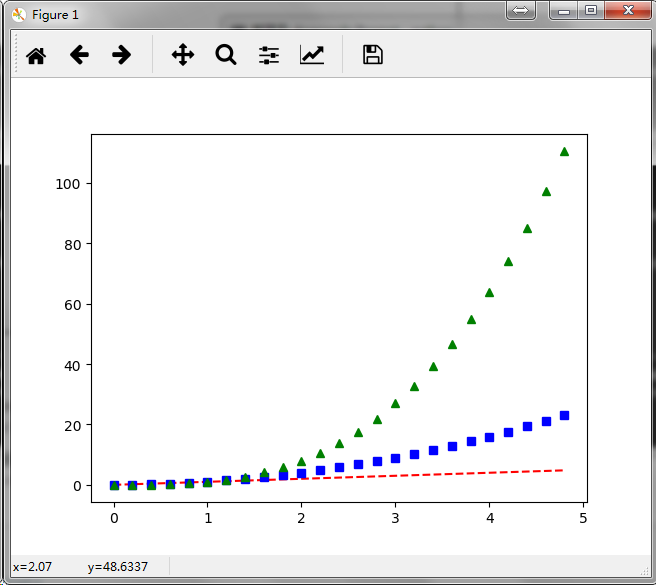补充：plot()  中，格式化字符串由颜色、标识符和线型三部分组成，定义格式如下：

fmt = '[color][marker][line]'

 character color 'b' blue 'g' green 'r' red 'c' cyan 'm' magenta 'y' yellow 'k' black 'w' white

 character description '.' point marker ',' pixel marker 'o' circle marker 'v' triangle_down marker '^' triangle_up marker '<' triangle_left marker '>' triangle_right marker '1' tri_down marker '2' tri_up marker '3' tri_left marker '4' tri_right marker 's' square marker 'p' pentagon marker '*' star marker 'h' hexagon1 marker 'H' hexagon2 marker '+' plus marker 'x' x marker 'D' diamond marker 'd' thin_diamond marker '|' vline marker '_' hline marker

 character description '-' solid line style '--' dashed line style '-.' dash-dot line style ':' dotted line style

以下是几个格式化字符串的示例：

'b'    # blue markers with default shape
'ro'   # red circles
'g-'   # green solid line
'--'   # dashed line with default color
'k^:'  # black triangle_up markers connected by a dotted line

# 使用关键字绘图

Matplotlib 允许用户提供带有关键字的数据对象作为参数，一旦提供了带有关键字的数据，就能够直接使用关键字来代替这些变量去生成图形。
我们可以使用某些命令来获取到这种格式的数据，例如：numpy.recarraypandas.DataFrame  。

data = {'a': np.arange(50),
'c': np.random.randint(0, 50, 50),
'd': np.random.randn(50)}
data['b'] = data['a'] + 10 * np.random.randn(50)
data['d'] = np.abs(data['d']) * 100

plt.scatter('a', 'b', c='c', s='d', data=data)
plt.xlabel('entry a')
plt.ylabel('entry b')
plt.show()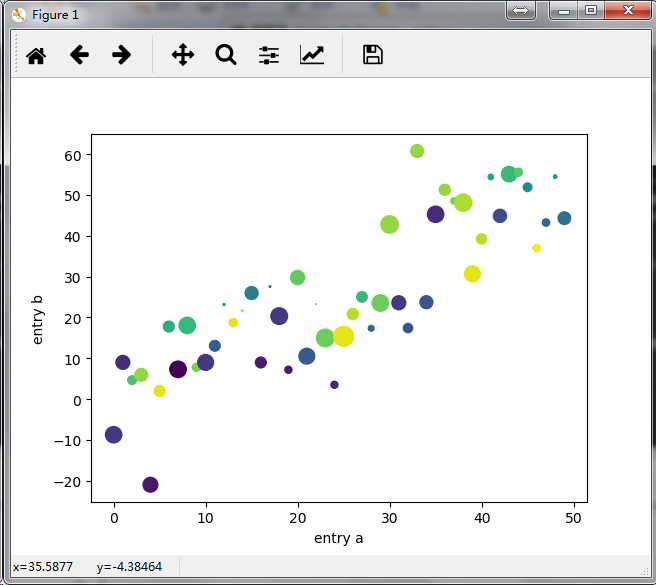# 使用分类变量绘图

names = ['group_a', 'group_b', 'group_c']
values = [1, 10, 100]

plt.figure(1, figsize=(9, 3))

plt.subplot(131)
plt.bar(names, values)
plt.subplot(132)
plt.scatter(names, values)
plt.subplot(133)
plt.plot(names, values)
plt.suptitle('Categorical Plotting')
plt.show()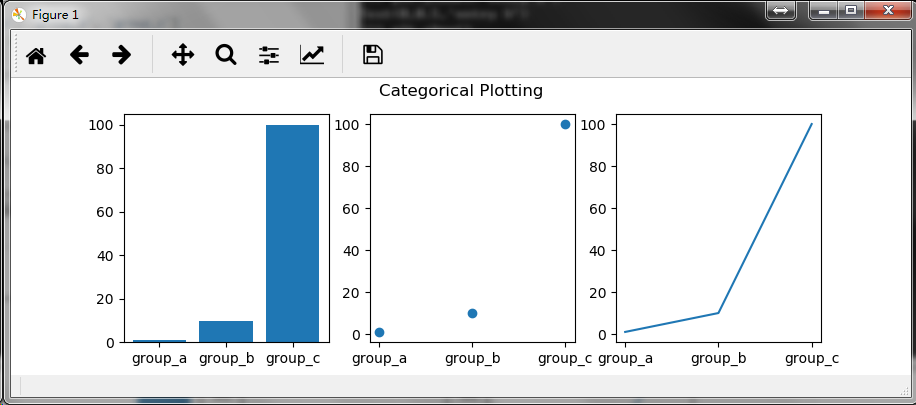# 控制线的属性

我们可以通过以下几种途径对线的属性进行设置：
使用关键字参数

plt.plot(x, y, linewidth=2.0)

plot() 命令会返回一个线对象的列表，例如：line1, line2 = plot(x1, y1, x2, y2)。在下面的代码中，我们将假设我们只有一条线即plot() 命令返回的线列表的长度为 1 。我们使用 line, 来获取列表中的第一个元素。

line, = plt.plot(x, y, '-')
line.set_antialiased(False) # turn off antialising

使用setp()命令
下面示例一下如何使用MATLAB风格的命令对一个线列表的多个属性进行设置。很明显地，setp() 能够作用于一个对象列表或者是一个单独的对象。你既可以使用Python 的关键字作为参数也可以使用MATLAB风格的string/value 对作为参数：

lines = plt.plot(x1, y1, x2, y2)
# use keyword args
plt.setp(lines, color='r', linewidth=2.0)
# or MATLAB style string value pairs
plt.setp(lines, 'color', 'r', 'linewidth', 2.0)

2D 线实例的可用属性见下表：

 Property Value Type alpha float animated [True | False] antialiased or aa [True | False] clip_box a matplotlib.transform.Bbox instance clip_on [True | False] clip_path a Path instance and a Transform instance, a Patch color or c any matplotlib color contains the hit testing function dash_capstyle ['butt' | 'round' | 'projecting'] dash_joinstyle ['miter' | 'round' | 'bevel'] dashes sequence of on/off ink in points data (np.array xdata, np.array ydata) figure a matplotlib.figure.Figure instance label any string linestyle or ls [ '-' | '--' | '-.' | ':' | 'steps' | ...] linewidth or lw float value in points lod [True | False] marker [ '+' | ',' | '.' | '1' | '2' | '3' | '4' ] markeredgecolor or mec any matplotlib color markeredgewidth or mew float value in points markerfacecolor or mfc any matplotlib color markersize or ms float markevery [ None | integer | (startind, stride) ] picker used in interactive line selection pickradius the line pick selection radius solid_capstyle ['butt' | 'round' | 'projecting'] solid_joinstyle ['miter' | 'round' | 'bevel'] transform a matplotlib.transforms.Transform instance visible [True | False] xdata np.array ydata np.array zorder any number

如果你想要获取可供设置的线属性的列表，你可以直接调用 setp() 命令并传入一条线或者传入一个线列表作为唯一参数。

lines = plt.plot([1, 2, 3])

plt.setp(lines)
alpha: float
animated: [True | False]
antialiased or aa: [True | False]
...snip

# 使用多图形和多轴

MATLAB和 pyplot 都有当前图形和当前轴的概念，所有的绘图命令都会被应用到当前轴上。在 Matplotlib 中，gca() 命令用来获取当前轴（一个 matplotlib.axes.Axes 实例），gcf() 命令用来获取当前图形（一个 matplotlib.figure.Figure 实例）。通常情况下，你并不需要考虑这些，因为 Matplotlib 会在后台对它们进行自动处理。
下面是一个创建了两个子图形的示例脚本：

def f(t):
return np.exp(-t) * np.cos(2*np.pi*t)

t1 = np.arange(0.0, 5.0, 0.1)
t2 = np.arange(0.0, 5.0, 0.02)

plt.figure(1)
plt.subplot(211)
plt.plot(t1, f(t1), 'bo', t2, f(t2), 'k')

plt.subplot(212)
plt.plot(t2, np.cos(2*np.pi*t2), 'r--')
plt.show()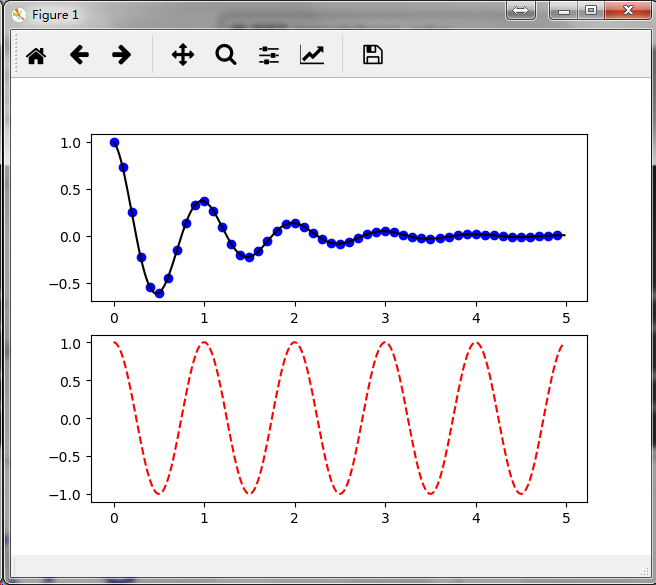figure()命令在这儿是一个可选项，因为默认创建的就是 figure(1) ，同样地，在没有手动地指定任何轴的情况下，将默认创建 subplot(111) 。subplot() 命令需要指定行数、列数、图形编号，其中图形编号的取值范围为（1 ~ 行数*列数）。如果行数*列数<10，参数之间的逗号可以省略。因此，subplot(211) 和 subplot(2, 1, 1) 的作用是一样的。

手动设置坐标系示例：https://matplotlib.org/gallery/subplots_axes_and_figures/axes_demo.html
绘制多个子图形示例：
https://matplotlib.org/gallery/subplots_axes_and_figures/subplot_demo.html
https://matplotlib.org/gallery/subplots_axes_and_figures/subplots_demo.html
你可以通过多次调用figure() 方法并传入一个递增的图形编号来创建多个图形。当然，只要你喜欢每一个图形也可以包含多个子图形或者坐标系：

import matplotlib.pyplot as plt
plt.figure(1)                # the first figure
plt.subplot(211)             # the first subplot in the first figure
plt.plot([1, 2, 3])
plt.subplot(212)             # the second subplot in the first figure
plt.plot([4, 5, 6])

plt.figure(2)                # a second figure
plt.plot([4, 5, 6])          # creates a subplot(111) by default

plt.figure(1)                # figure 1 current; subplot(212) still current
plt.subplot(211)             # make subplot(211) in figure1 current
plt.title('Easy as 1, 2, 3') # subplot 211 title
plt.show()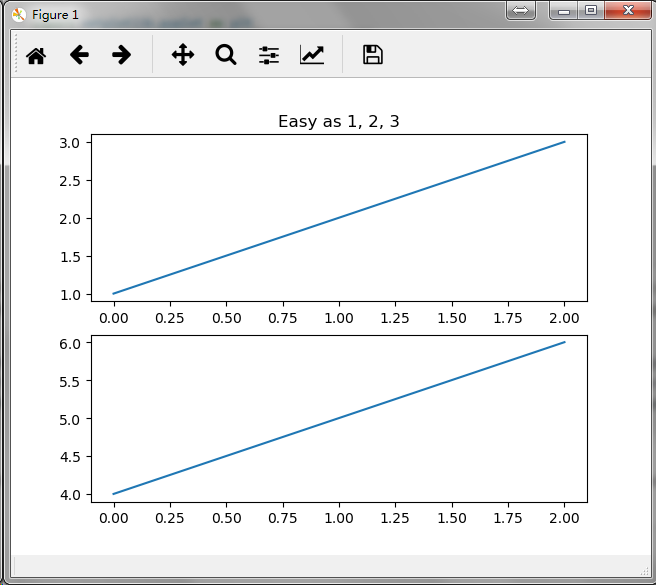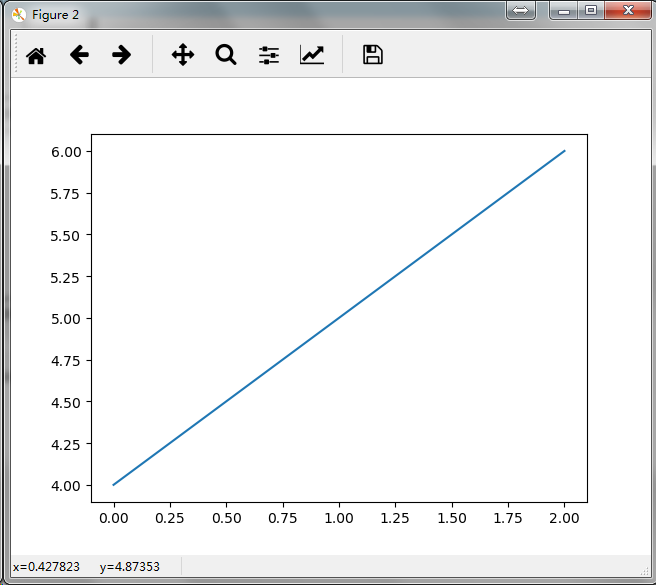你可以使用 clf() 命令来清除当前图形或者使用 cla() 命令来清除当前坐标系。 如果你觉得由后台帮你维护图形状态（指当前的图像，图形和坐标系）这种方式比较烦人，无须绝望：这只是对面向对象API的一个瘦状态封装，你可以把它替换掉（参见 Artist tutorial ）。
如果你是在创建多个图形，你还需要注意另外一件事：图形所占用的内存直到你调用 close() 显式地将其关闭才会全部释放，如果只删除掉图形的引用或者通过窗口管理器销毁掉屏幕上的图像展示窗口，图形所占用的内存是不能完全被释放的，因为pyplot 在 close() 命令被调用之前仍会对其内部引用进行维护。

# 使用文本

mu, sigma = 100, 15
x = mu + sigma * np.random.randn(10000)

# the histogram of the data
n, bins, patches = plt.hist(x, 50, density=1, facecolor='g', alpha=0.75)

plt.xlabel('Smarts')
plt.ylabel('Probability')
plt.title('Histogram of IQ')
plt.text(60, .025, r'$\mu=100,\ \sigma=15$')
plt.axis([40, 160, 0, 0.03])
plt.grid(True)
plt.show()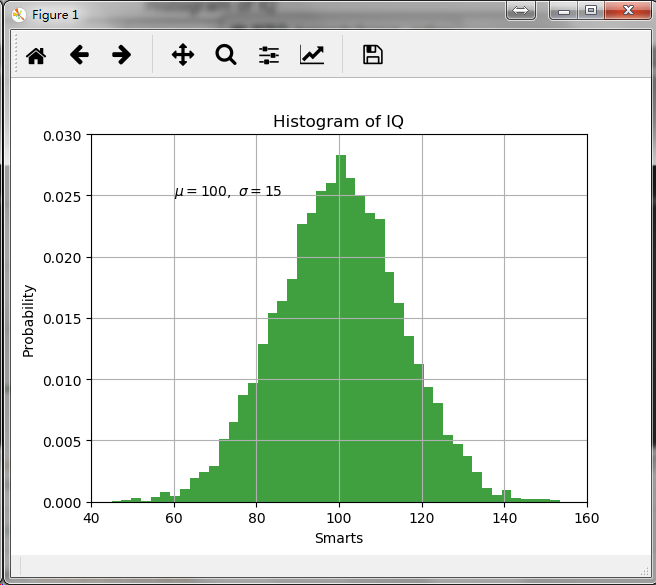t = plt.xlabel('my data', fontsize=14, color='red')

关于文本的属性的更多细节请参见：https://matplotlib.org/tutorials/text/text_props.html

# 在文本中使用数学表达式

Matplotlib 接收的文本表达式中可以包含 TeX 方程表达式，比如，书写时，需要使用 "$" 符号把TeX表达式包起来： plt.title(r'$\sigma_i=15\$')

Matplotlib 有一个內建的 TeX 方程式的语法分析器和布局引擎，并带有自己的数学字体（详情参见  Writing mathematical expressions ）。因此你可以不用安装TeX就能够跨平台使用数学表达式，对于那些已经安装了LaTeX和dvipng的用户，你也可以使用LaTex 来格式化你的文字并将输出的文本并入到你要展示的图形或者要保存的脚本中（详情参见 Text rendering With LaTeX）。

# 注释文本

ax = plt.subplot(111)

t = np.arange(0.0, 5.0, 0.01)
s = np.cos(2*np.pi*t)
line, = plt.plot(t, s, lw=2)

plt.annotate('local max', xy=(2, 1), xytext=(3, 1.5),
arrowprops=dict(facecolor='black', shrink=0.05),
)

plt.ylim(-2, 2)
plt.show()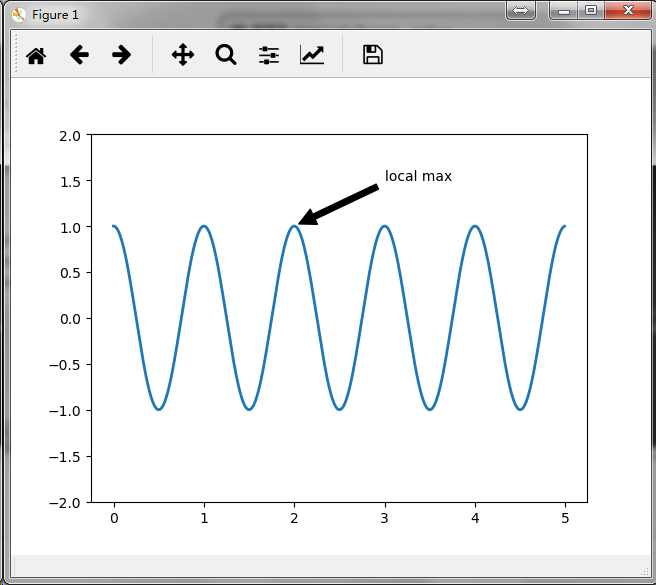# 对数及其他非线性坐标系

matplotlib.pylot 除了支持线性坐标系外，也支持对数和分对数等非线性坐标系，当数据的量级跨度比较大时会经常用到这些坐标系，改变一个坐标轴的刻度非常容易：

plt.xscale('log')

from matplotlib.ticker import NullFormatter  # useful for logit scale

# Fixing random state for reproducibility
np.random.seed(19680801)

# make up some data in the interval ]0, 1[
y = np.random.normal(loc=0.5, scale=0.4, size=1000)
y = y[(y > 0) & (y < 1)]
y.sort()
x = np.arange(len(y))

# plot with various axes scales
plt.figure(1)

# linear
plt.subplot(221)
plt.plot(x, y)
plt.yscale('linear')
plt.title('linear')
plt.grid(True)

# log
plt.subplot(222)
plt.plot(x, y)
plt.yscale('log')
plt.title('log')
plt.grid(True)

# symmetric log
plt.subplot(223)
plt.plot(x, y - y.mean())
plt.yscale('symlog', linthreshy=0.01)
plt.title('symlog')
plt.grid(True)

# logit
plt.subplot(224)
plt.plot(x, y)
plt.yscale('logit')
plt.title('logit')
plt.grid(True)
# Format the minor tick labels of the y-axis into empty strings with
# NullFormatter, to avoid cumbering the axis with too many labels.
plt.gca().yaxis.set_minor_formatter(NullFormatter())
# Adjust the subplot layout, because the logit one may take more space
# than usual, due to y-tick labels like "1 - 10^{-3}"
wspace=0.35)

plt.show()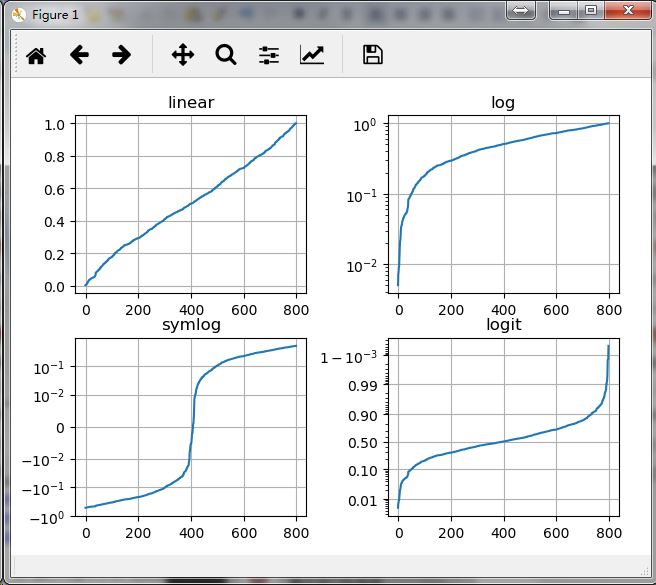08-2004-26
04-28
11-20387
11-172692
02-232287
05-059373
10-016045
10-1112万+
06-09225
10-25243
07-24849
07-30155
11-223万+
06-12557
11-2038
10-129万+点击重新获取扫码支付余额充值